# Texas Go Math Grade 7 Lesson 3.1 Answer Key Converting Between Measurement Systems

Refer to our Texas Go Math Grade 7 Answer Key Pdf to score good marks in the exams. Test yourself by practicing the problems from Texas Go Math Grade 7 Lesson 3.1 Answer Key Converting Between Measurement Systems.

## Texas Go Math Grade 7 Lesson 3.1 Answer Key Converting Between Measurement Systems

The table shows equivalencies between the customary and metric systems. You can use these equivalencies to convert a measurement ¡n one system to a measurement in the other system.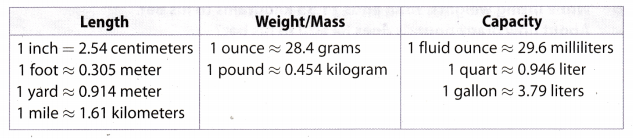Most conversions are approximate, as indicated by the symbol ≈.

The length of a sheet of paper is 11 inches. What is this length in centimeters?

A. You can use a bar diagram to solve this problem. Each part represents 1 inch.
1 inch ________ centimeter(s)C. 11 inches = ________ centimeters

Reflect

Question 1.
Communicate Mathematical Ideas Suppose you wanted to use a diagram to convert ounces to grams. Which unit would the parts in your diagram represent?
The parts in my diagram would represent ounces.

Lesson 3.1 Converting Between Measurement Systems Answer Key Question 2.
6 quarts ≈ ___________ liters
1 quart ≈ 0.946 liter.
Write the conversion factor as ratio: $$\frac{0.946 \text { liter }}{1 \text { quart }}$$
6 quarts × $$\frac{0.946 \text { liter }}{1 \text { quart }}$$ = 5.736 liters

Question 3.
14 feet ≈ ____________ meters
1 foot ≈ 0.305 meter.
Write the conversion factor as ratio: $$\frac{0.305 \text { meter }}{1 \text { foot }}$$
14 feet × $$\frac{0.305 \text { meter }}{1 \text { foot }}$$ ≈ 4.27 meters

Question 4.
255.6 grams ≈ ___________ ounces
1 ounce ≈ 28.4 grams
Write the conversion factor as ratio: $$\frac{1 \text { ounce }}{28.4 \text { grams }}$$
255.6 grams × $$\frac{1 \text { ounce }}{28.4 \text { grams }}$$ ≈ 9 ounce

Question 5.
7 liters ≈ __________ quarts
1 quart ≈ 0.946 liter.
Write the conversion factor as ratio: $$\frac{1 \text { quart }}{0.946 \text { liter }}$$
7 liters × $$\frac{1 \text { quart }}{0.946 \text { liter }}$$ ≈ 7.4 quarts

Reflect

Question 6.
Error Analysis Yolanda found the area of Bob’s driveway in square meters as shown. Explain why Yolanda’s answer is incorrect.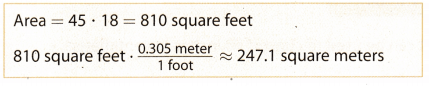Yolanda’s answer is incorrect because she multiplied square feet by a regular meter-to-foot ratio.

Converting within Measurement Systems Lesson 7.3 Answer Key Question 7.
A flower bed is 2 meters wide and 3 meters long. What is the area of the flower bed in square feet? Round intermediate steps and your answer to the nearest hundredth.
__________ square feet
First, convert flower bed measurements to feet
1 foot ≈ 0.305 meter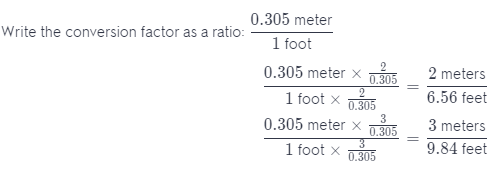Length ≈ 9.84 feet
Width ≈ 6.56 feet

Find the area.
Area = Length × Width
= 9.84 × 6.56
= 65.55 square feet

Complete each diagram to solve the problem. (Explore Activity)

Question 1.
Kate ran 5 miles. How far did she run in kilometers?
5 miles = __________________ kilometersFirst, complete the diagram. Then, with the help of a diagram convert units
5 × 1.61 = 8.05
5 miles ≈ 8.05 kilometres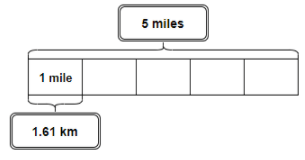Question 2.
Alex filled a 5-gallon jug with water. How many liters of water are in the container?
5 gallons ≈ _________________ litersFirst, complete the diagram. Then, with the help of a diagram convert units
5 × 3.79 = 18.95
5 gallons ≈ 18.95 litersUse a conversion factor to convert each measurement. (Example 1 and 2)

Question 3.
A ruler is 12 inches long. What is the length of this ruler in centimeters?
_________________ centimeters
1 in ≈ 2.54 centimeter.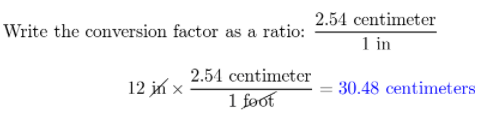The ruler is 30.48 centimeters long.

A kitten weighs 4 pounds. What is the approximate mass of the kitten?
_________________ kilograms
1 pound ≈ 0.454 kilograms
Write the conversion factor as a ratio: $$\frac{0.454 \text { kilograms }}{1 \text { pound }}$$
4 pounds × $$\frac{0.454 \text { kilograms }}{1 \text { pound }}$$ ≈ 1.816 kilogram
A kitten weighs 1.816 kilograms.

Use a proportion to convert each measurement. (Example 2)

Question 5.
20 yards ≈ ____________ meters
1 yard ≈ 0.914 meter.
Write the conversion factor as a ratio: $$\frac{0.914 \text { meter }}{1 \text { yard }}$$
20 yard × $$\frac{0.914 \text { meter }}{1 \text { yard }}$$ ≈ 18.28 meters

Question 6.
12 ounces ≈ ____________ grams
1 ounce ≈ 28.4 grams.
Write the conversion factor as a ratio: $$\frac{28.4 \text { grams }}{1 \text { ounce }}$$
12 ounces × $$\frac{28.4 \text { grams }}{1 \text { ounce }}$$ ≈ 340.8 meters

Question 7.
5 quarts ≈ ____________ liters
1 quart ≈ 0.946 grams.
Write the conversion factor as a ratio: $$\frac{0.946 \text { liter }}{1 \text { quart }}$$
5 quarts × $$\frac{0.946 \text { liter }}{1 \text { quart }}$$ ≈ 4.73 liters

Question 8.
400 meters ≈ ____________ yards
1 yard ≈ 0.914 meter.
Write the conversion factor as a ratio: $$\frac{1 \text { yard }}{0.914 \text { meter }}$$
400 meters × $$\frac{1 \text { yard }}{0.914 \text { meter }}$$ ≈ 437.64 yards

Question 9.
10 liters ≈ ____________ gallons
1 gallon ≈ 3.79 liters.
Write the conversion factor as a ratio: $$\frac{1 \text { gallon }}{3.79 \text { liters }}$$
10 liters × $$\frac{1 \text { gallon }}{3.79 \text { liters }}$$ ≈ 2.64 gallons

137.25 meters ≈ ____________ feet
1 foot ≈ 0.305 meters.
Write the conversion factor as a ratio: $$\frac{1 \text { foot }}{0.305 \text { meter }}$$
137.25 meters × $$\frac{1 \text { foot }}{0.305 \text { meter }}$$ ≈ 450 feet

Question 11.
165 centimeters ≈ ___________ inches
1 in = 2.54 centimeters.
Write the conversion factor as a ratio: $$\frac{1 \text { in }}{2.54 \text { centimeters }}$$
165 centimeters × $$\frac{1 \text { in }}{2.54 \text { centimeters }}$$ = 64.96 inches

Question 12.
10,000 kilometers ≈ ___________ miles
1 mile ≈ 1.61 kilometer.
Write the conversion factor as a ratio: $$\frac{1 \text { mile }}{1.61 \text { kilometer }}$$
10,000 kilometers × $$\frac{1 \text { mile }}{1.61 \text { kilometer }}$$ ≈ 6211.18 miles

Essential Question Check-In

Question 13.
Write and solve a proportion that can be used to convert 60 inches to centimeters.
1 in = 2.54 centimeters.Tell which measure is greater.

Question 14.
Six feet or two meters ________________
1 foot ≈ 0.305 meter.
Write the conversion factor as a ratio: $$\frac{1 \text { foot }}{0.305 \text { meter }}$$
2 meters × $$\frac{1 \text { foot }}{0.305 \text { meter }}$$ ≈ 6.56 feet

6.56 feet > 6 feet
2 meters > 6 feet

Question 15.
One inch or one centimeter ____________
1 in = 254 centimeters.
Notice it is obvious that one inch > one centimeter. There is no need for conversion.

One yard or one meter ____________
1 yard ≈ 0.9 14 meters.
Notice it is obvious that one meter > one yard. There is no need for conversion.

Question 17.
One mile or one kilometer ______________
1 mile ≈ 1.61 kilometer.
Notice it is obvious that one mile > one kilometer. There is no need for conversion.

Question 18.
One ounce or one gram ______________
1 ounce ≈ 28.4 grams.
Notice it is obvious that one ounce > one gram. There is no need for conversion.

Question 19.
One quart or one liter _________________
1 quart ≈ 0.946 liter.
Notice it is obvious that one liter > one quart. There is no need for conversion.

Question 20.
10 pounds or 10 kilograms ____________
1 pound ≈ 0.454 kiLograms.
Notice that it is the same problem as comparing 1 pound to 1 kilogram, it is obvious that one kilogram > one pound There is no need for conversion.

Question 21.
Four liters or one gallon ______________
1 gallon ≈ 3.79 liters.
4 liters > 3.79 liters
4 liters > 1 gallon

Question 22.
Two miles or three kilometers ___________
1 mile ≈ 1.61 kilometer
Write the conversion factor as a ratio: $$\frac{1 \text { mile }}{1.61 \text { kilometer }}$$
3 kilometer × $$\frac{1 \text { mile }}{1.61 \text { kilometer }}$$ ≈ 1.86 miles
2 miles > 1.86 miles
2 miles > 3 kilometers

Question 23.
What is the limit in kilograms?1 pound ≈ 0.454 kilogram
Write the conversion factor as a ratio: $$\frac{0.454 \text { kilogram }}{1 \text { pound }}$$
50 pounds × $$\frac{0.454 \text { kilogram }}{1 \text { pound }}$$ ≈ 22.7 kilogram
The limit is 22.7 kilograms.

Question 24.
What is the limit in miles per hour?1 mile per hour ≈ 1.61 kilometers per hour.
Write the conversion factor as a ratio: $$\frac{1 \text { mile per hour }}{1.61 \text { kilometer per hour }}$$
55 kilometers per hour × $$\frac{1 \text { mile per hour }}{1.61 \text { kilometer per hour }}$$ ≈ 34.16 miles per hour

Which container holds more, a half-gallon milk jug or a 2-liter juice bottle?
1 gallon ≈ 3.79 liters
Write the conversion factor as a ratio: $$\frac{1 \text { gallon }}{3.79 \text { liters }}$$
2 liters × $$\frac{1 \text { gallon }}{3.79 \text { liters }}$$ ≈ 0.53 gallon
0.53 gallon > 0.5 gallon
2 liters > 0.5 gallon
A 2-liter juice bottle contains more.

Question 26.
The label on a can of lemonade gives the volume as 12 fl oz,, or 355 mL. Verify that these two measurements are nearly equivalent.
1 fl oz ≈ 29.6 mL.
Write the conversion factor as a ratio: $$\frac{1 \mathrm{fl} \mathrm{oz}}{29.6 \mathrm{~mL}}$$
355 mL × $$\frac{1 \mathrm{fl} \mathrm{oz}}{29.6 \mathrm{~mL}}$$ ≈ 11.99 fl oz
12 fl oz ≈ 11.99 fl oz

Question 27.
The mass of a textbook is about 1.25 kilograms. About how many pounds is this?
1 pound ≈ 0.054 kilogram.
Write the conversion factor as a ratio: $$\frac{1 \text { pound }}{0.454 \text { kilogram }}$$
1.25 kilograms × $$\frac{1 \text { pound }}{0.454 \text { kilogram }}$$ ≈ 2.75 pounds
The mass of a textbook is about 2.75 pounds.

Question 28.
Critique Reasoning Michael estimated his mass as 8 kilograms. Is his estimate reasonable? Justify your answer.
No, his estimate is not reasonable. If he is at the age where he is capable of estimating his own weight in kilograms, then he must be at least 12 years old. Thus, he surely weighs ≥ 35 kilograms.

Question 29.
Your mother bought a three-liter bottle of water. When she got home, she discovered a small leak in the bottom and asked you to find a container to transfer the water into, All you could find were two half-gallon jugs.
a. Will your containers hold all of the water?
1 gallon ≈ 3.79 Liters.
Two half gallon = 1 gallon.
3.79 liters> 3 liters
1 gallon > 3 liters
The containers will hold all the water.

b. What If? Suppose an entire liter of water leaked out in the car. In that case, would you be able to fit all of the remaining water into one of the half-gallon jugs? Explain.
$$\frac{1}{2}$$ gallon = $$\frac{3.79}{2}$$ liters
= 1.895 liters
If one liter leaked out that means there is two liters left
2 Liters > 1.895 liters
We would not be able to fit the remaining water into half-gallon jug

Question 30.
The track team ran a mile and a quarter during their practice.
How many kilometers did the team run? ____________________________
1 mile ≈ 1.61 kilometer.
Write the conversion factor as a ratio: $$\frac{1.61 \text { kilometer }}{1 \mathrm{mile}}$$
1$$\frac{1}{4}$$ mile × $$\frac{1.61 \text { kilometer }}{1 \mathrm{mile}}$$ ≈ 2.0125 kilometers
The team ran 2.0125 kilometers.

Question 31.
A countertop is 16 feet long and 3 feet wide.
a. What is the area of the counter top in square meters? ______________ square meters
1 foot ≈ 0.305 meter.
Convert both length and width to meters.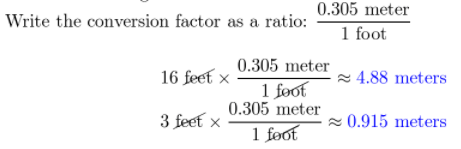Now, calculate the area.
A = length × width
= 4.88 × 0.915
= 4.47 m2

b. Tile costs $28 per square meter. How much will it cost to cover the countertop with new tile?$ ___________________________________
Multiply the cost per square meter by the area of the countertop in square meter.
28 × 4.47 = \$125.16

Question 32.
At a school picnic, your teacher asks you to mark a field every ten yards so students can play football. The teacher accidentally gave you a meter stick instead of a yardstick. How far apart in meters should you mark the lines if you still want them to be in the right places?
1 yard ≈ 0.914 meters.
We have to convert 10 yards to meters.
Write the conversion factor as a ratio: $$\frac{0.914 \text { meter }}{1 \text { yard }}$$
10 yards × $$\frac{0.914 \text { meter }}{1 \text { yard }}$$ ≈ 9.14 meters
We should mark the line every 9.14 meters.

Question 33.
You weigh a gallon of 2% milk in science class and learn that it is approximately 8.4 pounds. You pass the milk to the next group, and then realize that your teacher wanted an answer in kilograms, not pounds. Explain how you can adjust your answer without weighing the milk again. Then give the weight in kilograms.
1 pound ≈ 0.454 kiLograms.
We have to convert 10 yards to meters.
Write the conversion factor as a ratio: $$\frac{0.454 \text { kilograms }}{1 \text { pound }}$$
8.4 pounds × $$\frac{0.454 \text { kilograms }}{1 \text { pound }}$$ ≈ 3.81 kilogram
A gallon of 2% milk weighs 3.81 kilograms.

H.O.T. Focus On Higher Order Thinking

Question 34.
Analyze Relationships Annalisa, Keiko, and Stefan want to compare their heights. Annalisa is 64 inches tall. Stefan tells her, “I’m about 7.5 centimeters taller than you.” Keiko knows she is 1.5 inches shorter than Stefan. Give the heights of all three people in both inches and centimeters to the nearest half-unit.
First, convert Aniia1in’s height to centimeters to find out how tail Stefan is. Then, convert Stefan’s height to inches to find out how tall Keiko is.
All rounded to the nearest half.
1 in = 2.54 centimeters.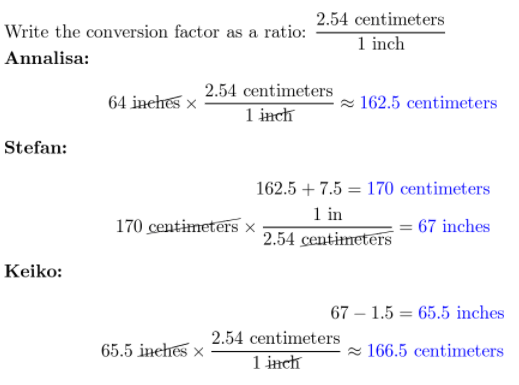Question 35.
Communicate Mathematical Ideas Mikhael wanted to rewrite the conversion factor” 1 yard 0.914 meters” to create a conversion factor to convert meters to yards. He wrote “1 meter ≈ ___________.” Tell how Mikhael should finish his conversion, and explain how you know.
Write the conversion factor as a ratio: $$\frac{0.914 \text { meter }}{1 \text { yard }}$$
1 meter × $$\frac{1 \text { yard }}{0.914 \text { meter }}$$ ≈ 1.09 yards